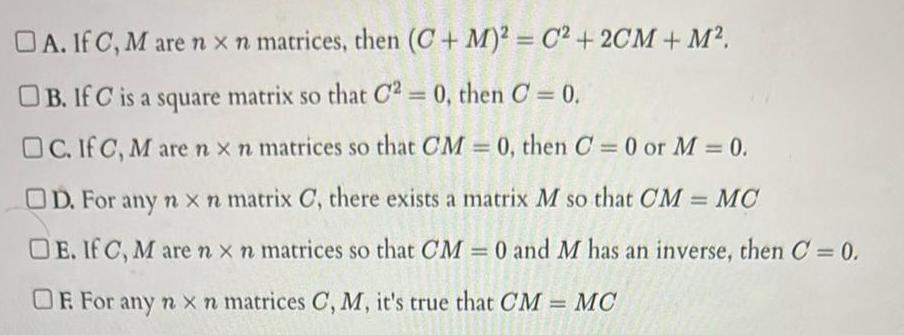Question:

# DA If C M are n x n matrices then C M C 2CM M B If C is a

Last updated: 11/13/2023DA If C M are n x n matrices then C M C 2CM M B If C is a square matrix so that C2 0 then C 0 OC If C Mare n x n matrices so that CM 0 then C 0 or M 0 OD For any n x n matrix C there exists a matrix M so that CM MC DE If C Mare nx n matrices so that CM 0 and M has an inverse then C 0 OF For any n x n matrices C M it s true that CM MC